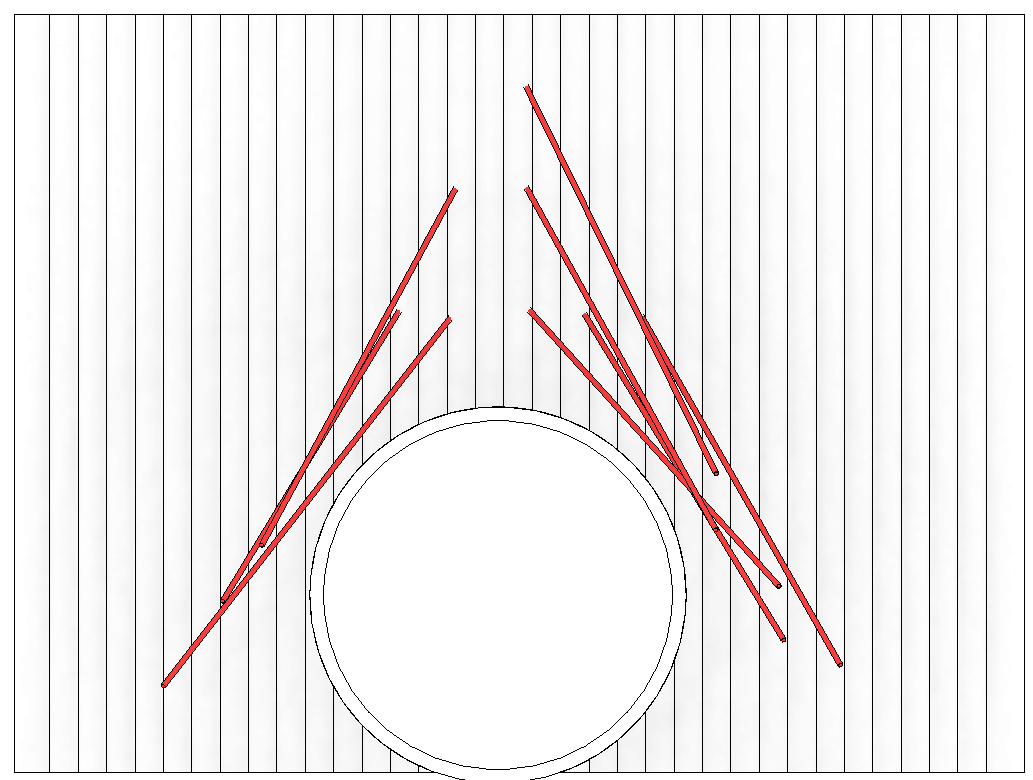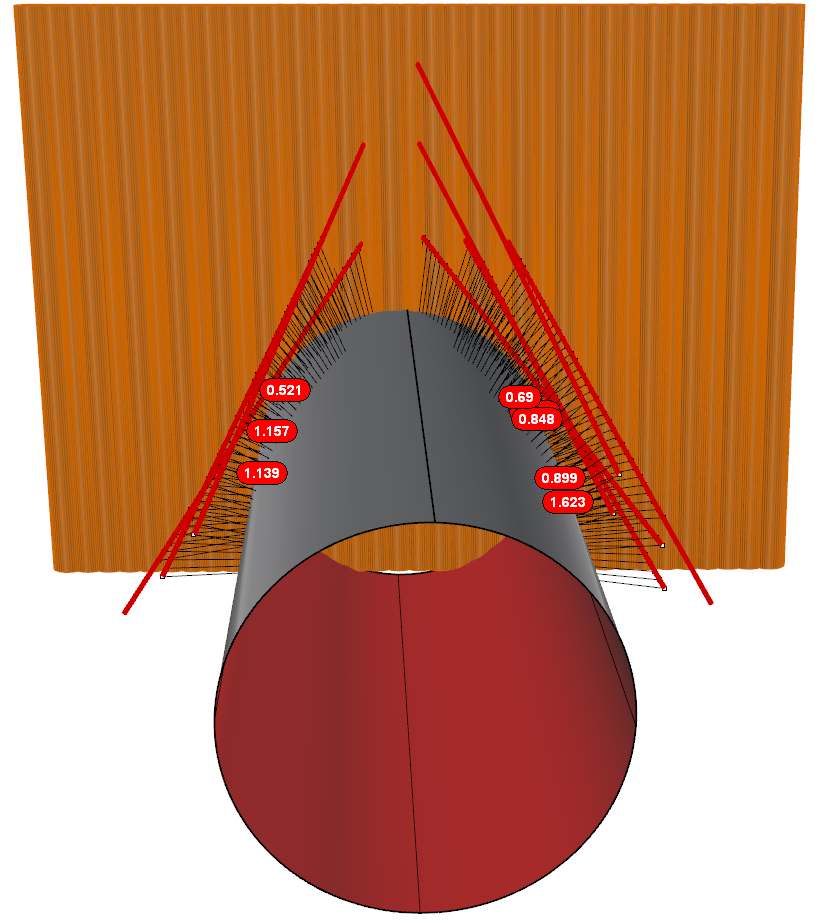# Minimum distance between curves and surface

I have modelled some anchors in Revit and I need to check the minimum distance between them and the tunnel external surface.This can be easily done exporting the model in Rhino and using a Python script.

``````import rhinoscriptsyntax as rs
surface_id = rs.GetObject("select surface", 8, True)
curve_id = rs.GetObject("select curve", 4)
points = rs.DivideCurve(curve_id, 50)
def evaluatedeviation(surface_id, threshold, sample):
r2point = rs.SurfaceClosestPoint(surface_id, sample)
r3point = rs.EvaluateSurface(surface_id, r2point, r2point)
deviation = rs.Distance(r3point, sample)
if deviation >= threshold: return